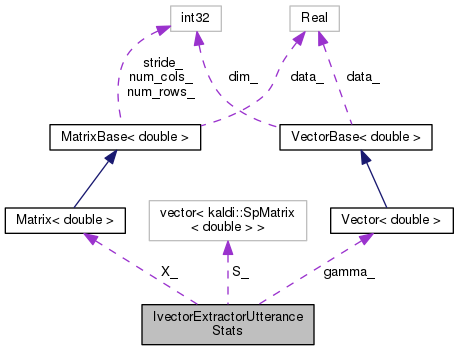IvectorExtractorUtteranceStats Class Reference

These are the stats for a particular utterance, i.e. More...

`#include <ivector-extractor.h>`

Collaboration diagram for IvectorExtractorUtteranceStats:[legend]

## Public Member Functions

IvectorExtractorUtteranceStats (int32 num_gauss, int32 feat_dim, bool need_2nd_order_stats)

void AccStats (const MatrixBase< BaseFloat > &feats, const Posterior &post)

void Scale (double scale)

double NumFrames ()

## Protected Attributes

Vector< double > gamma_

Matrix< double > X_

std::vector< SpMatrix< double > > S_

## Friends

class IvectorExtractor

class IvectorExtractorStats

## Detailed Description

These are the stats for a particular utterance, i.e.

the sufficient stats for estimating an iVector (if need_2nd_order_stats == true, we can also estimate the variance of the model; these 2nd order stats are not needed if we just need the iVector.

Definition at line 79 of file ivector-extractor.h.

## ◆ IvectorExtractorUtteranceStats()

 IvectorExtractorUtteranceStats ( int32 num_gauss, int32 feat_dim, bool need_2nd_order_stats )
inline

Definition at line 81 of file ivector-extractor.h.

References rnnlm::i.

82  :
83  gamma_(num_gauss), X_(num_gauss, feat_dim) {
84  if (need_2nd_order_stats) {
85  S_.resize(num_gauss);
86  for (int32 i = 0; i < num_gauss; i++)
87  S_[i].Resize(feat_dim);
88  }
89  }
kaldi::int32 int32
std::vector< SpMatrix< double > > S_

## ◆ AccStats()

 void AccStats ( const MatrixBase< BaseFloat > & feats, const Posterior & post )

Definition at line 852 of file ivector-extractor.cc.

854  {
855  typedef std::vector<std::pair<int32, BaseFloat> > VecType;
856  int32 num_frames = feats.NumRows(),
857  num_gauss = X_.NumRows(),
858  feat_dim = feats.NumCols();
859  KALDI_ASSERT(X_.NumCols() == feat_dim);
860  KALDI_ASSERT(feats.NumRows() == static_cast<int32>(post.size()));
861  bool update_variance = (!S_.empty());
862  SpMatrix<double> outer_prod(feat_dim);
863  for (int32 t = 0; t < num_frames; t++) {
864  SubVector<BaseFloat> frame(feats, t);
865  const VecType &this_post(post[t]);
866  if (update_variance) {
867  outer_prod.SetZero();
869  }
870  for (VecType::const_iterator iter = this_post.begin();
871  iter != this_post.end(); ++iter) {
872  int32 i = iter->first; // Gaussian index.
873  KALDI_ASSERT(i >= 0 && i < num_gauss &&
874  "Out-of-range Gaussian (mismatched posteriors?)");
875  double weight = iter->second;
876  gamma_(i) += weight;
878  if (update_variance)
880  }
881  }
882 }
MatrixIndexT NumCols() const
Returns number of columns (or zero for empty matrix).
Definition: kaldi-matrix.h:67
kaldi::int32 int32
const SubVector< Real > Row(MatrixIndexT i) const
Return specific row of matrix [const].
Definition: kaldi-matrix.h:188
std::vector< SpMatrix< double > > S_
#define KALDI_ASSERT(cond)
Definition: kaldi-error.h:185
MatrixIndexT NumRows() const
Returns number of rows (or zero for empty matrix).
Definition: kaldi-matrix.h:64

## ◆ NumFrames()

 double NumFrames ( )
inline

Definition at line 96 of file ivector-extractor.h.

Referenced by kaldi::TestIvectorExtraction().

96 { return gamma_.Sum(); }
Real Sum() const
Returns sum of the elements.

## ◆ Scale()

 void Scale ( double scale )

Definition at line 884 of file ivector-extractor.cc.

References rnnlm::i, and OnlineIvectorEstimationStats::Scale().

884  {
885  gamma_.Scale(scale);
886  X_.Scale(scale);
887  for (size_t i = 0; i < S_.size(); i++)
888  S_[i].Scale(scale);
889 }
void Scale(Real alpha)
Multiply each element with a scalar value.
std::vector< SpMatrix< double > > S_
void Scale(Real alpha)
Multiplies all elements by this constant.

## ◆ IvectorExtractor

 friend class IvectorExtractor
friend

Definition at line 99 of file ivector-extractor.h.

## ◆ IvectorExtractorStats

 friend class IvectorExtractorStats
friend

Definition at line 100 of file ivector-extractor.h.

## ◆ gamma_

 Vector gamma_
protected

## ◆ S_

 std::vector > S_
protected

Definition at line 103 of file ivector-extractor.h.

## ◆ X_

 Matrix X_
protected

Definition at line 102 of file ivector-extractor.h.

The documentation for this class was generated from the following files: